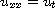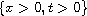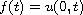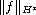Electron. J. Diff. Equ., Vol. 2015 (2015), No. 267, pp. 1-14.

### Stable algorithm for identifying a source in the heat equation Lahcene Chorfi, Leila Alem

Abstract:
We consider an inverse problem for the heat equationin the quarter planewhere one wants to determine the temperaturefrom the measured data. This problem is severely ill-posed and has been studied before. It is well known that the central difference approximation in time has a regularization effect, but the backward difference scheme is not well studied in theory and in practice. In this paper, we revisit this method to provide a stable algorithm. Assuming an a priori bound onwe derive a Holder type stability result. We give some numerical examples to show the efficiency of the proposed method. Finally, we compare our method to one based on the central or forward differences.

Submitted June 27, 2015. Published October 16, 2015.
Math Subject Classifications: 35K05, 65M32, 65T50.
Key Words: Inverse problem; heat equation; fourier regularization; finite difference.

Show me the PDF file (307 KB), TEX file, and other files for this article.Lahcène Chorfi Laboratoire de Mathématiques Appliquées Université B. M. d'Annaba B.P. 12, 23000 Annaba, Algérie email: l_chorfi@hotmail.com Leïla Alem Laboratoire de Mathématiques Appliquées Université B. M. d'Annaba B.P. 12, 23000 Annaba, Algérie email: alemleila@yahoo.fr